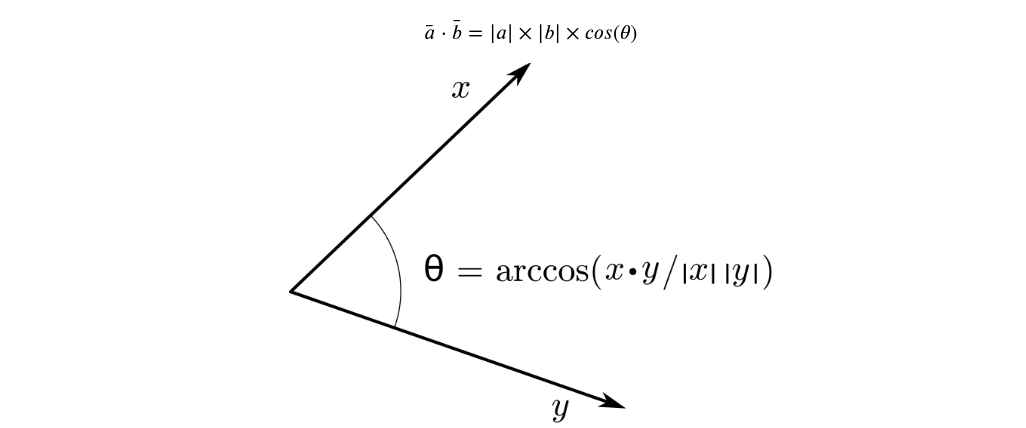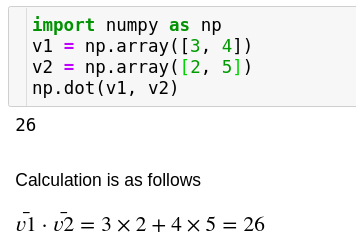1598863800

# Advanced Operations Using Numpy Arrays

In my previous post, I talk about Reduction Operations in Numpy Arrays. You may read through it before you move on to the more Advanced Operations below.

The topics covered in this post are as follows:

You can click on any of these above to jump to the respective section.

## Introduction

Numpy consists of a subpackage called linalg which has functions particularly pertaining to linear algebra which is an integral part in the working of many DL and ML algorithms. We will discuss several concepts about these operations along with their numpy implementation which will inevitably become a part of your Data Science toolkit. We will be covering three of the most important operations that can be carried out with numpy arrays which are heavily used in DL and ML applications such as Natural Language Processing, Image Retrieval tasks and Customer Recommendation tasks. Without any further delay, let’s get started!

## The Dot Product

In vector algebra, the dot product represnts a scalar quantity obtained by sum aggregating the product of vectors along the n-dimensional space respectively.

In linear algebra, dot product is typically used for finding out if two vectors are perpendicular to each other or to find out the magnitude of a single vector or to find out the projection of a vector along another vector.

In Data Science, the dot product is typically used to find out the similarity or distance between two or more vectors in some high dimensional space. When we perform nearest neighbour search this is what we typically use. The similarity found out using dot product is called cosine similarity because the dot product theoretically is given by the expressionThe smaller the angle between the two vectors, more closely aligned the two vectors are since the cosine of an angle is high when the angle itself is small/low.#programming #numpy #python #arrays #data-science

## Buddha Community1595467140

## NumPy Array Tutorial - Python NumPy Array Operations and Methods

The most important feature of NumPy is the homogeneous high-performance n-dimensional array object. Data manipulation in Python is nearly equivalent to the manipulation of NumPy arrays. NumPy array manipulation is basically related to accessing data and sub-arrays. It also includes array splitting, reshaping, and joining of arrays. Even the other external libraries in Python relate to NumPy arrays.

_Keeping you updated with latest technology trends, _Join DataFlair on Telegram

## Numpy Array Basics

Arrays in NumPy are synonymous with lists in Python with a homogenous nature. The homogeneity helps to perform smoother mathematical operations. These arrays are mutable. NumPy is useful to perform basic operations like finding the dimensions, the bite-size, and also the data types of elements of the array.

## NumPy Array Creation

### 1. Using the NumPy functions

NumPy has a variety of built-in functions to create an array.

#### a. Creating one-dimensional array in NumPy

For 1-D arrays the most common function is np.arange(…), passing any value create an array from 0 to that number.

1. import numpy as np
2. array=np.arange(20)
3. array

Output

array([ 0, 1, 2, 3, 4, 5, 6, 7, 8, 9, 10, 11,12, 13, 14, 15, 16, 17, 18, 19])

We can check the dimensions by using array.shape.

#numpy tutorials #array in numpy #numpy array #python numpy array1595235180

## NumPy Features - Why we should use Numpy?

Welcome to DataFlair!!! In this tutorial, we will learn Numpy Features and its importance.

NumPy is a library for the Python programming language, adding support for large, multi-dimensional arrays and matrices, along with a large collection of high-level mathematical functions to operate on these arrays

NumPy (Numerical Python) is an open-source core Python library for scientific computations. It is a general-purpose array and matrices processing package. Python is slower as compared to Fortran and other languages to perform looping. To overcome this we use NumPy that converts monotonous code into the compiled form.#### NumPy Features

These are the important features of NumPy:

#### 1. High-performance N-dimensional array object

This is the most important feature of the NumPy library. It is the homogeneous array object. We perform all the operations on the array elements. The arrays in NumPy can be one dimensional or multidimensional.

#### a. One dimensional array

The one-dimensional array is an array consisting of a single row or column. The elements of the array are of homogeneous nature.

#### b. Multidimensional array

In this case, we have various rows and columns. We consider each column as a dimension. The structure is similar to an excel sheet. The elements are homogenous.

#### 2. It contains tools for integrating code from C/C++ and Fortran

We can use the functions in NumPy to work with code written in other languages. We can hence integrate the functionalities available in various programming languages. This helps implement inter-platform functions.

#numpy tutorials #features of numpy #numpy features #why use numpy #numpy1595235240

## NumPy Applications - Uses of Numpy

In this Numpy tutorial, we will learn Numpy applications.

NumPy is a basic level external library in Python used for complex mathematical operations. NumPy overcomes slower executions with the use of multi-dimensional array objects. It has built-in functions for manipulating arrays. We can convert different algorithms to can into functions for applying on arrays.NumPy has applications that are not only limited to itself. It is a very diverse library and has a wide range of applications in other sectors. Numpy can be put to use along with Data Science, Data Analysis and Machine Learning. It is also a base for other python libraries. These libraries use the functionalities in NumPy to increase their capabilities.#### 1. An alternative for lists and arrays in Python

Arrays in Numpy are equivalent to lists in python. Like lists in python, the Numpy arrays are homogenous sets of elements. The most important feature of NumPy arrays is they are homogenous in nature. This differentiates them from python arrays. It maintains uniformity for mathematical operations that would not be possible with heterogeneous elements. Another benefit of using NumPy arrays is there are a large number of functions that are applicable to these arrays. These functions could not be performed when applied to python arrays due to their heterogeneous nature.

#### 2. NumPy maintains minimal memory

Arrays in NumPy are objects. Python deletes and creates these objects continually, as per the requirements. Hence, the memory allocation is less as compared to Python lists. NumPy has features to avoid memory wastage in the data buffer. It consists of functions like copies, view, and indexing that helps in saving a lot of memory. Indexing helps to return the view of the original array, that implements reuse of the data. It also specifies the data type of the elements which leads to code optimization.

#### 3. Using NumPy for multi-dimensional arrays

We can also create multi-dimensional arrays in NumPy.These arrays have multiple rows and columns. These arrays have more than one column that makes these multi-dimensional. Multi-dimensional array implements the creation of matrices. These matrices are easy to work with. With the use of matrices the code also becomes memory efficient. We have a matrix module to perform various operations on these matrices.

#### 4. Mathematical operations with NumPy

Working with NumPy also includes easy to use functions for mathematical computations on the array data set. We have many modules for performing basic and special mathematical functions in NumPy. There are functions for Linear Algebra, bitwise operations, Fourier transform, arithmetic operations, string operations, etc.

#numpy tutorials #applications of numpy #numpy applications #uses of numpy #numpy1595500395

## NumPy Bitwise Operators with Examples

NumPy includes a package to perform bitwise operations on the array elements. These NumPy bitwise operators perform bit by bit operations. It performs the function of two-bit values to produce a new value. There are functions to convert the elements into their binary representation and then apply operations on the bits.#### NumPy Bitwise Operators

This is a specific package that applies bitwise operations on the binary format of elements. These functions compare the binary value of elements and then produce output. There are 6 basic bitwise operations available in NumPy

1. bitwise_and()- It calculates the bit-wise AND operation between two array elements.

2. bitwise_or()- It calculates the bit-wise OR operation between two array elements.

3. invert()- It calculates the bit-wise NOT operation between two array elements.

4. bitwise_xor()- It calculates the bit-wise OR operation between two array elements.

5. left_shift()- This operator shifts the bits of the binary representation of the element towards left.

6. right_shift()- This operator shifts the bits of the binary representation of the element towards the right.

#### 1. Bitwise AND operator

The function performs bitwise AND on two array elements. The bitwise function performs an operation on the corresponding bits of the binary representation of the operands i.e. elements. The output of the operation depends on the AND truth table. If both the corresponding values are 1 only then the output will be 1, otherwise 0. Here 1 can also is equivalent to True and 0 as False. Hence the result will be True only if both the values are True, otherwise, it will result to be False.

#numpy tutorials #numpy binary operators #numpy bitwise operators #numpy1619660285

## Linear Algebra for Data Scientists with NumPy - Analytics India Magazine

A geek in Machine Learning with a Master’s degree in…How NumPy becomes the base of Data Science computing system (source)